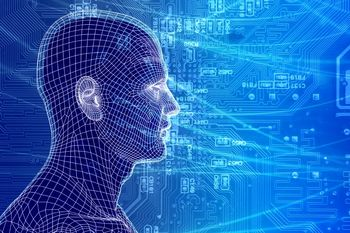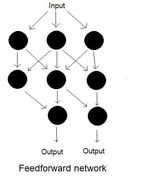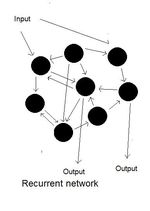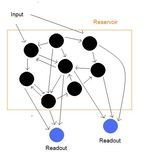# Reservoir computing: A New Hope?Artificial neural networks are computational models inspired by the organization of neurons in the brain. They are used to model and analyze data, to implement algorithms and in attempts to understand the computational principles used by the brain. The popularity of neural networks in computer science, machine learning and cognitive science has varied wildly, both across time and between people. To an enthusiast, neural networks are seen as a revolutionary way of conceiving of computation; the entry point to robust, distributed, easily parallelizable processing; the means to build artificial intelligence systems that replicate the complexity of the brain; and a way to understand the computations that the brain carries out. To skeptics they are poorly understood and over-hyped, offering little insight into general computational principles either in computer science or in cognition. Neural networks are often called “the second best solution to any problem”. Depending on where you stand, this either means that they are often promising but never actually useful or that they are applicable to a range of problems and do almost as well as solutions explicitly tailored to the particular details of a problem (and only applicable to that particular problem).

Neural networks typically consist of a number of simple information processing units (the “neurons”). Each neuron combines a number of inputs (some or all of which come from other neurons) to give an output, which is then typically used as input to other neurons in the network. The connections between neurons normally have weights, which determine the strength of the effect of the neurons on each other. So, for example, a simple neuron could sum up all its inputs weighted by the connection strengths and give an output of 0 or 1 depending on whether this sum is below or above some threshold. This output then functions as an input to other neurons, with appropriate weights for each connection.

A computation involves transforming some stream of input into some stream of output. For example, the input stream might be a list of numbers that come into the network one by one, and the desired output stream might be the squares of those numbers. Some or all of the neurons receive the input through connections just like those between neurons. The output stream is taken to be the output of some particular set of neurons in the network. The network can be programmed to do a particular transformation (“trained”) by adjusting the strengths of connections between different neurons and between the inputs and the neurons. Typically this is done before the network is used to process the desired input, but sometimes the connection weights are changed according to some pre-determined rule as the network processes input.

Training a network by modifying the connection weights between elements is quite different from programming a computer, which involves combining a series of simple operations on data to get a desired output. In general, training a network is quite hard. Changing a particular connection strength changes the behavior of the whole network in a way that isn't easily deduced from just the two neurons participating in the connection. Similarly, once the network has been trained, it's hard to interpret the role of an individual weight in the overall computation. Indeed, the popularity of neural networks has waxed and waned with advances in algorithms for training and interpreting connection weights.One way to make this problem tractable is to use a simpler class of network. A “feedforward” network is one in which neurons do not form loops, and information flows in only one direction. So if neuron A connects to neuron B, and neuron B connects to neuron C, then neuron C can't connect to neuron A. Feedforward networks have the advantage that a change in a weight only affects a certain subset of neurons (the neurons downstream of the connection) and the effect of the change on the output is easy to understand. This makes training feedforward networks comparatively simple and algorithms to do this have been around for a while.However, using just feedforward networks is quite restrictive. “Recurrent” networks (networks that allow loops) are able to maintain memories, make it easier to combine information that entered the network at different times and make it easier for an input signal to be transformed in different ways; they are also more like the networks seen in the cortex, which makes them better candidates for understanding the brain. Overcoming the barriers to training recurrent networks is thus quite valuable.

Reservoir computing is a new approach to constructing neural networks that tries to combine several of the useful features of recurrent networks with a feedforward-like ease of training. The typical network here consists of a large recurrent network (the “reservoir”), which receives the input and a smaller “readout” circuit, which receives connections from some or all of the neurons in the recurrent network but doesn't connect back to them. The connection weights in the recurrent network are fixed when the network is constructed and then don't change. Reservoir computing is based on a pair of insights, each of which is more or less relevant in different contexts.To understand the first, imagine a memory task. You give a recurrent network an input signal for a while and then stop, requiring the network to retain a memory of that input signal so that it can be used at some later time. For example, you want to give your network a number and then have it output the square of that number at some time in the future; more realistically, imagine some circuit in your brain trying to keep track of a phone number until you can find a pen and paper.

The standard way to do this is to have the signal push the network into a state where the outputs of the neurons activate each other leading to an unchanging steady-state (for example, a representation of the sequence of digits in the phone number). However, even if there are no stable states in the network (besides the one where no neuron is active), the network still has some memory. An input will excite certain neurons and then bounce around the loops in the network before fading away. A simple example would be neuron A activating neuron B, which activates neuron C, which activates neuron A and so on, progressively losing strength. As long as these traces fade away slowly enough, and as long as they can be distinguished from the traces of other signals, the system has a useful memory. This approach gives rise to the names under which reservoir networks were first introduced: “echo-state networks” and “liquid-state machines”. The signal “echoes” around the reservoir as it slowly dies out and these echoes are used for computation; alternately, instead of echoes, the traces are like the ripples on the surface of a pond after a weight has been dropped in. At least for a while, you can reconstruct where the weight was dropped in.

More generally, instead of giving the network an input signal, stopping the input signal and then having the network reproduce it, you could give the network a continuous stream of input and require it to convert this to a stream of output, where the output at any given time depends on the previous history of the input. In this case, the echoes of the signal at previous times would combine with the signal at the current time in the network. For many networks, the history of the input can still be extracted (i.e. the echoes can be separated). Building a network that has useful echoes is much easier than building one with a particular set of stable states: you can pick connection weights randomly from a large class of distributions and the network will have this property.

The other insight exploits the fact that recurrent networks are more complex than feedforward ones and can more easily transform an input signal into a diversity of output signals. Traditionally the weights in a recurrent network would be chosen to implement a particular transformation but, as previously discussed, this is often hard to do. Instead, you can use the fact that having a large (but not absurdly large) number of neurons and choosing the weights randomly gives you a diverse set of transformations. Each neuron is effectively computing some transformation of the input signal (depending on how it's connected) and, if the echoes are strong enough, some of these transformations depend on the history of the input as well as the current input state. In general, none of these is the transformation you want to compute but, if you've created a diverse reservoir of transformations, some of them can be combined to give you the transformation you want.

This is where the previously mentioned readout unit comes in. Since the readout does not connect back into the reservoir, the connections between the reservoir and the readout are feedforward. The weights of these connections are now changed so that the readout approximates the desired transformation by combining the outputs of the reservoir. The calculation done by the readout is simple but it uses the complex transformations calculated by the reservoir as building blocks. Calculating the weights for the readout is a simple calculation, since it involves no loops (remember that the weights in the reservoir, which involve loops, do not change), and, as long as the reservoir has enough memory and is implementing a diverse set of transformations, the readout should do well. Also note that the same recurrent network can be used to calculate multiple transformations in parallel, as long as they have separate readouts.

There are a few different properties this reservoir needs to have. If you give it an input signal and then stop, the input needs to bounce around for long enough to make memory-based computation feasible. On the other hand, if signals bounce around for too long then the echoes from different signals will be hard to distinguish from each other. Simultaneously the recurrent network needs to be able to compute a diverse set of transformations of the input signal. The initial set of fixed weights given to the recurrent network will affect these properties, and studying how to choose these weights for particular applications is an active area of research. But it is driven by the initial discovery that choosing the weights randomly is often quite effective and that, in general, choosing a good reservoir is much simpler than explicitly trying to train the recurrent network to do particular transformations.

Reservoir computing is only a few years old, but has created a lot of interest as a promising way to exploit recurrent networks for computational purposes while still preserving the tractability of a feedforward network. Mathematicians and computer scientists are interested in it for its possible applications to problems in pattern recognition and prediction, and are working on characterizing what makes a good reservoir and when a reservoir-based approach is most likely to succeed. Neuroscientists are looking for evidence that the brain uses these sorts of networks. Given the boom-and-bust history of fads in neural networks, it's probably too early to make any grand pronouncements. Still, at the very least, reservoir computing provides an interesting new way of thinking about how to compute with networks and how to compute in parallel, both of which are long-standing scientific problems.

 Feedforward networks can be specifically designed to give you some limited memory, but this isn't a general property.

 The architecture of a feedforward network doesn't have to be too complex in order to approximate all reasonable transformations but this usually requires extremely large numbers of neurons.

 Reservoir computing was introduced by Jaeger H. (2001) and Maass W., Natschlaeger T. and Markram H. (2002)

 The weights do not need to be chosen randomly. You just need a diversity of transformations and it turns out that this can often be achieved without any special structure in the weights. This is linked to the observation that, in high dimensions, randomly chosen sets often do nearly as well as optimally chosen sets for certain tasks (and certain meanings of “random”).

 To some extent this property includes the previous one: having a diversity of transformations often means having some with long memory. This is equivalent to having the “echoes” of the signal lasting for a long time in the network.